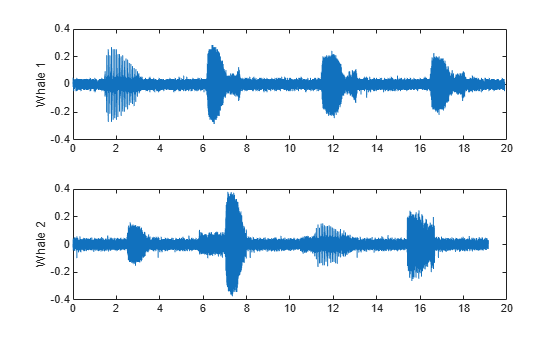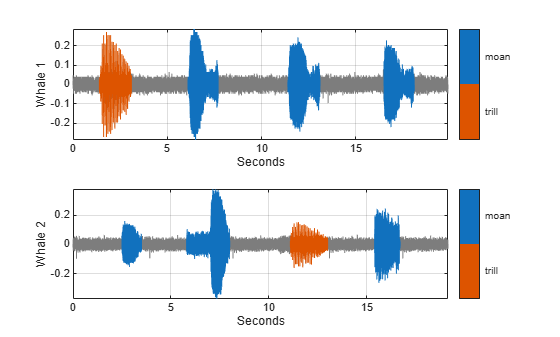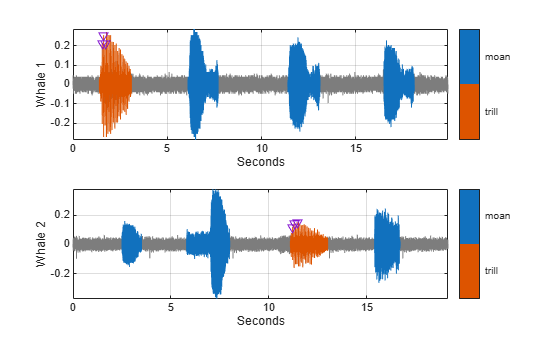# labeledSignalSet

Create labeled signal set

## Description

Use `labeledSignalSet` to store labeled signals along with the label definitions. Create signal label definitions using `signalLabelDefinition`.

## Creation

### Syntax

``lss = labeledSignalSet``
``lss = labeledSignalSet(src)``
``lss = labeledSignalSet(src,lbldefs)``
``lss = labeledSignalSet(src,lbldefs,'MemberNames',mnames)``
``lss = labeledSignalSet(src,lbldefs,Name,Value)``

### Description

````lss = labeledSignalSet` creates an empty labeled signal set. Use `addMembers` to add signals to the set. Use `addLabelDefinitions` to add label definitions to the set.```
````lss = labeledSignalSet(src)` creates a labeled signal set for the input data source `src`. Use `addLabelDefinitions` to add label definitions to the set.```
````lss = labeledSignalSet(src,lbldefs)` creates a labeled signal set for the input data source `src` using the signal label definitions `lbldefs`. Use `signalLabelDefinition` to create signal label definitions.```
````lss = labeledSignalSet(src,lbldefs,'MemberNames',mnames)` creates a labeled signal set for the input data source `src` and specifies names for the members of the set. Use `setMemberNames` to modify the member names. `lbldefs` is optional.```

example

````lss = labeledSignalSet(src,lbldefs,Name,Value)` sets Properties using name-value pairs. You can specify multiple name-value pairs. Enclose each property name in quotes. `lbldefs` is optional.```

### Input Arguments

expand all

Input data source, specified as a matrix, a cell array, a timetable, a `signalDatastore` object, or an `audioDatastore` (Audio Toolbox) object. `src` implicitly specifies the number of members of the set, the number of signals in each member, and the data in each signal.

Example: `{randn(10,3),randn(17,9)}` has two members. The first member contains three 10-sample signals. The second member contains nine 17-sample signals.

Example: `{{randn(10,1)},{randn(17,1),randn(27,1)}}` has two members. The first member contains one 10-sample signal. The second member contains a 17-sample signal and a 27-sample signal.

Example: `{{timetable(seconds(1:10)',randn(10,3)),timetable(seconds(1:7)',randn(7,2))},{timetable(seconds(1:3)',randn(3,1))}}` has two members. The first member contains three signals sampled at 1 Hz for 10 seconds and two signals sampled at 1 Hz for 7 seconds. The second member contains one signal sampled at 1 Hz for 3 seconds.

#### Example: `signalDatastore` Object Pointing to Files

Specify the path to a set of sample sound signals included as MAT-files with MATLAB®. Each file contains a signal variable and a sample rate. List the names of the files.

```folder = fullfile(matlabroot,"toolbox","matlab","audiovideo"); lst = dir(append(folder,"/*.mat")); nms = {lst(:).name}'```
```nms = 7x1 cell {'chirp.mat' } {'gong.mat' } {'handel.mat' } {'laughter.mat'} {'mtlb.mat' } {'splat.mat' } {'train.mat' } ```

Create a signal datastore that points to the specified folder. Set the sample rate variable name to `Fs`, which is common to all files. Generate a subset of the datastore that excludes the file `mtlb.mat`, which differs from the other files in that the signal variable is not called `y`.

```sds = signalDatastore(folder,"SampleRateVariableName","Fs"); sdss = subset(sds,~strcmp(nms,"mtlb.mat"));```

Use the subset datastore as the source for a `labeledSignalSet` object.

`lss = labeledSignalSet(sdss)`
```lss = labeledSignalSet with properties: Source: [1x1 signalDatastore] NumMembers: 6 TimeInformation: "inherent" Labels: [6x0 table] Description: "" Use labelDefinitionsHierarchy to see a list of labels and sublabels. Use setLabelValue to add data to the set. ```

Label definitions, specified as a vector of `signalLabelDefinition` objects.

Member names, specified as a character vector, a string scalar, a cell array of character vectors, or a string array.

Example: ```labeledSignalSet({randn(100,1) randn(10,1)},'MemberNames',{'llama' 'alpaca'})``` specifies a set of random signals with two members, `'llama'` and `'alpaca'`.

## Properties

expand all

Labeled signal set description, specified as a character vector or string scalar.

Example: ```'Description','Sleep test patients by sex and age'```

Data Types: `char` | `string`

Sample rate values, specified as a positive scalar or a vector. This property is valid only when the data source does not contain inherent time information.

• Set `SampleRate` to a positive numeric scalar to specify the same sample rate for all signals in the labeled set.

• Set `SampleRate` to a vector to specify that each member of the labeled set has signals sampled at the same rate, but the sample rates differ from member to member. The vector must have a number of elements equal to the number of members of the set. If a member of a set has signals with different sample rates, then specify the sample rates using timetables.

Example: `'SampleRate',[1e2 1e3]` specifies that the signals in the first member of a set are sampled at a rate of 100 Hz and the signals in the second member are sampled at 1 kHz.

Sample time values, specified as a positive scalar, a vector, a `duration` scalar, or a `duration` vector. This property is valid only when the data source does not contain inherent time information.

• Set `SampleTime` to a numeric or `duration` scalar to specify the same sample time for all signals in the labeled set.

• Set `SampleTime` to a numeric or `duration` vector to specify that each member of the labeled set has signals with the same time interval between samples, but the intervals differ from member to member. The vector must have a number of elements equal to the number of members of the set. If a member of a set has signals with different sample times, then specify the sample times using timetables.

Example: `'SampleTime',seconds([1e-2 1e-3])` specifies that the signals in the first member of a set have 0.01 second between samples, and the signals in the second member have 1 millisecond between samples.

Time values, specified as a vector, a `duration` vector, a matrix, or a cell array. This property is valid only when the data source does not contain inherent time information. Time values must be unique and increasing.

• Set `TimeValues` to a numeric or `duration` vector to specify the same time values for all signals in the labeled set. The vector must have the same length as all the signals in the set.

• Set `TimeValues` to a numeric or `duration` matrix or cell array to specify that each member of the labeled set has signals with the same time values, but the time values differ from member to member.

• If `TimeValues` is a matrix, then it must have a number of columns equal to the number of members of the set. All signals in the set must have a length equal to the number of rows of the matrix.

• If `TimeValues` is a cell array, then it must contain a number of vectors equal to the number of members of the set. All signals in a member must have a length equal to the number of elements of the corresponding vector in the cell array.

If a member of a set has signals with different time values, then specify the time values using timetables.

Example: `'TimeValues',[1:1000;0:1/500:2-1/500]'` specifies that the signals in the first member of a set are sampled 1 Hz for 1000 seconds. The signals in the second member are sampled at 500 Hz for 2 seconds.

Example: `'TimeValues',seconds([1:1000;0:1/500:2-1/500]')` specifies that the signals in the first member of a set are sampled 1 Hz for 1000 seconds. The signals in the second member are sampled at 500 Hz for 2 seconds.

Example: `'TimeValues',{1:1000,0:1/500:2-1/500}` specifies that the signals in the first member of a set are sampled 1 Hz for 1000 seconds. The signals in the second member are sampled at 500 Hz for 2 seconds.

Example: `'TimeValues',{seconds(1:1000),seconds(0:1/500:2-1/500)}` specifies that the signals in the first member of a set are sampled 1 Hz for 1000 seconds. The signals in the second member are sampled at 500 Hz for 2 seconds.

Number of members in set, specified as a positive integer.

Labels table, specified as a MATLAB® table. Each variable of `Labels` corresponds to a label defined for the set. Each row of `Labels` corresponds to a member of the data source. The row names of `Labels` are the member names.

Data Types: `table`

Time information of source, specified as one of the following:

• `'none'` — The signals in the source have no time information.

• `'sampleRate'` — The signals in the source are sampled at a specified rate.

• `'sampleTime'` — The signals in the source have a specified time interval between samples.

• `'timeValues` — The signals in the source have a time value corresponding to each sample.

• `'inherent'` — The signals in the source contain inherent time information. MATLAB timetables are an example of such signals.

Data Types: `char` | `string`

Data source of labeled signal set, specified as a matrix, a timetable, a cell array, or an audio datastore.

• If `Source` is a numeric matrix, then the labeled signal set has one member that contains a number of signals equal to the number of matrix columns.

Example: `labeledSignalSet(randn(10,3))` has one member that contains three 10-sample signals.

• If `Source` is a cell array of matrices, then the labeled signal set has a number of members equal to the number of matrices in the cell array. Each member contains a number of signals equal to the number of columns of the corresponding matrix.

Example: `labeledSignalSet({randn(10,3),randn(17,9)})` has two members. The first member contains three 10-sample signals. The second member contains nine 17-sample signals.

• If `Source` is a cell array, and each element of the cell array is a cell array of numeric vectors, then the labeled signal set has a number of members equal to the number of cell array elements. Each signal within a member can have any length.

Example: `labeledSignalSet({{randn(10,1)},{randn(17,1),randn(27,1)}})` has two members. The first member contains one 10-sample signal. The second member contains a 17-sample signal and a 27-sample signal.

• If `Source` is a timetable with variables containing numeric values, then the labeled signal set has one member that contains a number of signals equal to the number of variables. The time values of the timetable must be of type `duration`, unique, and increasing.

Example: `labeledSignalSet(timetable(seconds(1:10)',randn(10,3)))` has one member that contains three signals sampled at 1 Hz for 10 seconds.

• If `Source` is a cell array of timetables, and each timetable has an arbitrary number of variables with numeric values, then the labeled signal set has a number of members equal to the number of timetables. Each member contains a number of signals equal to the number of variables in the corresponding timetable.

Example: `labeledSignalSet({timetable(seconds(1:10)',randn(10,3)),timetable(seconds(1:5)',randn(5,13))})` has two members. The first member contains three signals sampled at 1 Hz for 10 seconds. The second member contains 13 signals sampled at 1 Hz for 5 seconds.

• If `Source` is a cell array, and each element of the cell array is a cell array of timetables, then the labeled signal set has a number of members equal to the number of cell array elements. Each member can have any number of timetables, and each timetable within a member can have any number of variables.

Example: `labeledSignalSet({{timetable(seconds(1:10)',randn(10,3)),timetable(seconds(1:7)',randn(7,2))},{timetable(seconds(1:3)',randn(3,1))}})` has two members. The first member contains three signals sampled at 1 Hz for 10 seconds and two signals sampled at 1 Hz for 7 seconds. The second member contains one signal sampled at 1 Hz for 3 seconds.

• If the input data source, `src`, is an audio datastore, then the labeled signal set has a number of members equal to the number of files to which the datastore points. The `Source` property contains a cell array of character vectors with the file names. Each member contains all the signals returned by the read of the corresponding datastore file.

## Object Functions

 `addLabelDefinitions` Add label definitions to labeled signal set `addMembers` Add members to labeled signal set `editLabelDefinition` Edit label definition properties `getLabelDefinitions` Get label definitions in labeled signal set `getLabeledSignal` Get labeled signals from labeled signal set `getLabelNames` Get label names in labeled signal set `getLabelValues` Get label values from labeled signal set `getMemberNames` Get member names in labeled signal set `getSignal` Get signals from labeled signal set `head` Get top rows of labels table `labelDefinitionsHierarchy` Get hierarchical list of label and sublabel names `labelDefinitionsSummary` Get summary table of signal label definitions `merge` Merge two or more labeled signal sets `removeLabelDefinition` Remove label definition from labeled signal set `removeMembers` Remove members from labeled signal set `removePointValue` Remove row from point label `removeRegionValue` Remove row from ROI label `resetLabelValues` Reset labels to default values `setLabelValue` Set label value in labeled signal set `setMemberNames` Set member names in labeled signal set `subset` Get new labeled signal set with subset of members

## Examples

collapse all

Consider a set of whale sound recordings. The recorded whale sounds consist of trills and moans. Trills sound like series of clicks. Moans are low-frequency cries similar to the sound made by a ship's horn. You want to look at each signal and label it to identify the whale type, the trill regions, and the moan regions. For each trill region, you also want to label the signal peaks higher than a certain threshold.

Signal Label Definitions

Define an attribute label to store whale types. The possible categories are blue whale, humpback whale, and white whale.

```dWhaleType = signalLabelDefinition('WhaleType',... 'LabelType','attribute',... 'LabelDataType','categorical',... 'Categories', ["blue" "humpback" "white"],... 'Description','Whale type'); ```

Define a region-of-interest (ROI) label to capture moan regions. Define another ROI label to capture trill regions.

```dMoans = signalLabelDefinition('MoanRegions',... 'LabelType','roi',... 'LabelDataType','logical',... 'Description','Regions where moans occur'); dTrills = signalLabelDefinition('TrillRegions',... 'LabelType','roi',... 'LabelDataType','logical',... 'Description','Regions where trills occur'); ```

Finally, define a point label to capture the trill peaks. Set this label as a sublabel of the `dTrills` definition.

```dTrillPeaks = signalLabelDefinition('TrillPeaks',... 'LabelType','point',... 'LabelDataType','numeric',... 'Description','Trill peaks'); dTrills.Sublabels = dTrillPeaks;```

Labeled Signal Set

Create a `labeledSignalSet` with the whale signals and the label definitions. Add label values to identify the whale type, the moan and trill regions, and the peaks of the trills.

```load labelwhalesignals lbldefs = [dWhaleType dMoans dTrills]; lss = labeledSignalSet({whale1 whale2},lbldefs,'MemberNames',{'Whale1' 'Whale2'}, ... 'SampleRate',Fs,'Description','Characterize wave song regions'); ```

Visualize the label hierarchy and label properties using `labelDefinitionsHierarchy` and `labelDefinitionsSummary`.

`labelDefinitionsHierarchy(lss)`
```ans = 'WhaleType Sublabels: [] MoanRegions Sublabels: [] TrillRegions Sublabels: TrillPeaks ' ```
`labelDefinitionsSummary(lss)`
```ans=3×9 table LabelName LabelType LabelDataType Categories ValidationFunction DefaultValue Sublabels Tag Description ______________ ___________ _____________ ____________ __________________ ____________ ___________________________ ___ ____________________________ "WhaleType" "attribute" "categorical" {3x1 string} {["N/A" ]} {0x0 double} {0x0 double } "" "Whale type" "MoanRegions" "roi" "logical" {["N/A" ]} {0x0 double} {0x0 double} {0x0 double } "" "Regions where moans occur" "TrillRegions" "roi" "logical" {["N/A" ]} {0x0 double} {0x0 double} {1x1 signalLabelDefinition} "" "Regions where trills occur" ```

The signals in the loaded data correspond to songs of two blue whales. Set the `'WhaleType'` values for both signals.

```setLabelValue(lss,1,'WhaleType','blue'); setLabelValue(lss,2,'WhaleType','blue');```

Visualize the `'Labels'` property. The table has the newly added `'WhaleType'` values for both signals.

`lss.Labels `
```ans=2×3 table WhaleType MoanRegions TrillRegions _________ ___________ ____________ Whale1 blue {0x2 table} {0x3 table} Whale2 blue {0x2 table} {0x3 table} ```

Visualize Region Labels

Visualize the whale songs to identify the trill and moan regions.

```subplot(2,1,1) plot((0:length(whale1)-1)/Fs,whale1) ylabel('Whale 1') subplot(2,1,2) plot((0:length(whale2)-1)/Fs,whale2) ylabel('Whale 2')```Moan regions are sustained low-frequency wails.

• `whale1` has moans centered at about 7 seconds, 12 seconds, and 17 seconds.

• `whale2` has moans centered at about 3 seconds, 7 seconds, and 16 seconds.

Add the moan regions to the labeled set. Specify the ROI limits in seconds and the label values.

```moanRegionsWhale1 = [6.1 7.7; 11.4 13.1; 16.5 18.1]; mrsz1 = [size(moanRegionsWhale1,1) 1]; setLabelValue(lss,1,'MoanRegions',moanRegionsWhale1,true(mrsz1)); moanRegionsWhale2 = [2.5 3.5; 5.8 8; 15.4 16.7]; mrsz2 = [size(moanRegionsWhale2,1) 1]; setLabelValue(lss,2,'MoanRegions',moanRegionsWhale2,true(mrsz2));```

Trill regions have distinct bursts of sound punctuated by silence.

• `whale1` has a trill centered at about 2 seconds.

• `whale2` has a trill centered at about 12 seconds.

Add the trill regions to the labeled set.

```trillRegionWhale1 = [1.4 3.1]; trsz1 = [size(trillRegionWhale1,1) 1]; setLabelValue(lss,1,'TrillRegions',trillRegionWhale1,true(trsz1)); trillRegionWhale2 = [11.1 13]; trsz2 = [size(trillRegionWhale1,1) 1]; setLabelValue(lss,2,'TrillRegions',trillRegionWhale2,true(trsz2));```

Create a `signalMask` object for each whale song and use it to visualize and label the different regions. For better visualization, change the label values from logical to categorical.

```mr1 = getLabelValues(lss,1,'MoanRegions'); mr1.Value = categorical(repmat("moan",mrsz1)); tr1 = getLabelValues(lss,1,'TrillRegions'); tr1.Value = categorical(repmat("trill",trsz1)); msk1 = signalMask([mr1;tr1],'SampleRate',Fs); subplot(2,1,1) plotsigroi(msk1,whale1) ylabel('Whale 1') hold on mr2 = getLabelValues(lss,2,'MoanRegions'); mr2.Value = categorical(repmat("moan",mrsz2)); tr2 = getLabelValues(lss,2,'TrillRegions'); tr2.Value = categorical(repmat("trill",trsz2)); msk2 = signalMask([mr2;tr2],'SampleRate',Fs); subplot(2,1,2) plotsigroi(msk2,whale2) ylabel('Whale 2') hold on```Visualize Point Labels

Label three peaks for each trill region. For point labels, you specify the point locations and the label values. In this example, the point locations are in seconds.

```peakLocsWhale1 = [1.553 1.626 1.7]; peakValsWhale1 = [0.211 0.254 0.211]; setLabelValue(lss,1,["TrillRegions" "TrillPeaks"],... peakLocsWhale1,peakValsWhale1,'LabelRowIndex',1); subplot(2,1,1) plot(peakLocsWhale1,peakValsWhale1,'v') hold off peakLocsWhale2 = [11.214 11.288 11.437]; peakValsWhale2 = [0.119 0.14 0.15]; setLabelValue(lss,2,["TrillRegions" "TrillPeaks"],... peakLocsWhale2,peakValsWhale2,'LabelRowIndex',1); subplot(2,1,2) plot(peakLocsWhale2,peakValsWhale2,'v') hold off```Explore Label Values

Explore the label values using `getLabelValues`.

`getLabelValues(lss)`
```ans=2×3 table WhaleType MoanRegions TrillRegions _________ ___________ ____________ Whale1 blue {3x2 table} {1x3 table} Whale2 blue {3x2 table} {1x3 table} ```

Retrieve the moan regions for the first member of the labeled set.

`getLabelValues(lss,1,'MoanRegions')`
```ans=3×2 table ROILimits Value ____________ _____ 6.1 7.7 {} 11.4 13.1 {} 16.5 18.1 {} ```

Use a second output argument to list the sublabels of a label.

`[value,valueWithSublabel] = getLabelValues(lss,1,'TrillRegions')`
```value=1×2 table ROILimits Value __________ _____ 1.4 3.1 {} ```
```valueWithSublabel=1×3 table ROILimits Value Sublabels TrillPeaks __________ _____ ___________ 1.4 3.1 {} {3x2 table} ```

To retrieve the values in a sublabel, express the label name as a two-element array.

`getLabelValues(lss,1,["TrillRegions" "TrillPeaks"])`
```ans=3×2 table Location Value ________ __________ 1.553 {[0.2110]} 1.626 {[0.2540]} 1.7 {[0.2110]} ```

Find the value of the third trill peak corresponding to the second member of the set.

```getLabelValues(lss,2,["TrillRegions" "TrillPeaks"], ... 'LabelRowIndex',1,'SublabelRowIndex',3)```
```ans=1×2 table Location Value ________ __________ 11.437 {[0.1500]} ```